Question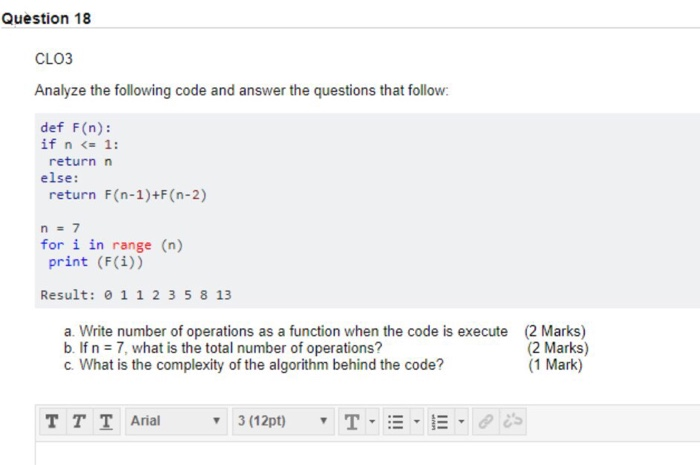Let, F(n) be the nth fibonacci number. F(0) = 0, F(1) = 1 and F(n) = F(n - 1) + F(n - 2) and say G(n) be the no. of operations or the function call of F(n) then G(0) = G(1) = 1 and it is derived that G(n) = 2 * F(n + 1) - 1 because here we considered F(0) = 0, F(1) = 1

a. For calculating F(0),F(1)...F(6) we need 2 * (1 + 1 + 2 + 3 + 5 + 8 + 13) - 7 = 59 operations

b. if n = 7 then we need 2 * F(8) - 1 = 2 * 21 - 1 = 41 operations.

c. Complexity of the algorithm is O(2^n) because at most two functions are being called in each function call.

#### Earn Coins

Coins can be redeemed for fabulous gifts.

Similar Homework Help Questions
• ### Question 4 CLO3 The following Python script implements an algorithm to find and prints the max value in a list of values. MAX 0 def MaxVal (Ist): for i in Ist: if( MAX < i): MAX = i return (MA...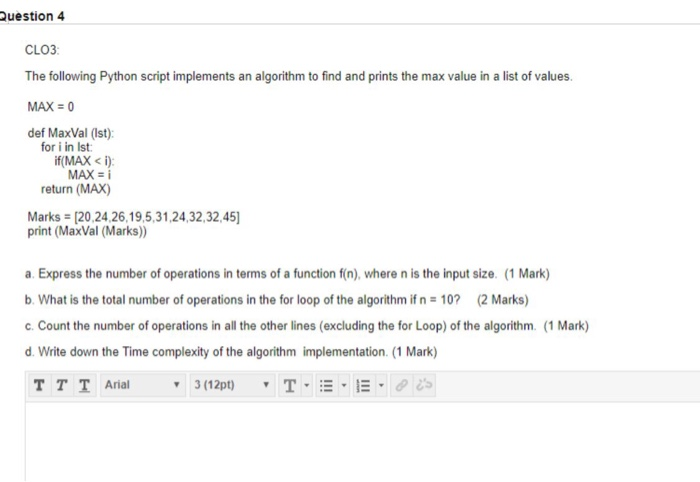Question 4 CLO3 The following Python script implements an algorithm to find and prints the max value in a list of values. MAX 0 def MaxVal (Ist): for i in Ist: if( MAX < i): MAX = i return (MAX) Marks (20,24,26,19,5,31,24,32,32,45 print (MaxVal (Marks) a. Express the number of operations in terms of a function f(n), where n is the input size. (1 Mark) b. What is the total number of operations in the for loop of the algorithm...

• ### def number(n): result = 1 for i in range(1, n+1): result = result * i return...

def number(n): result = 1 for i in range(1, n+1): result = result * i return result In the above code, what is the "parameter?"

• ### How to prove G（n）=n+1 in this algorithm？ 1. if (n 0) 2. return 1 3. else if (n1) f 4. return 2 5. else if (n 2) 6. return 3 7. else if (n3) t 8. return 4 else f 9. int OGnew int[n 11 10. G[O]1 1...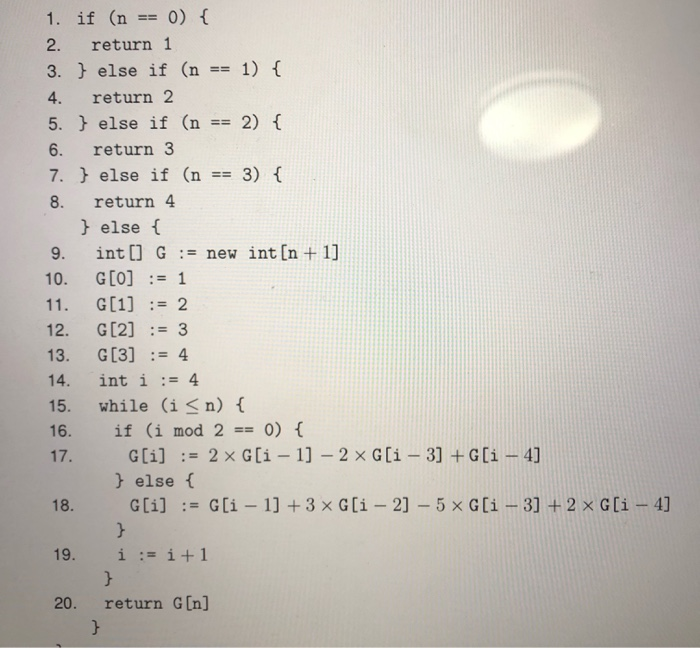How to prove G（n）=n+1 in this algorithm？ 1. if (n 0) 2. return 1 3. else if (n1) f 4. return 2 5. else if (n 2) 6. return 3 7. else if (n3) t 8. return 4 else f 9. int OGnew int[n 11 10. G[O]1 12. G3 13. G4 14. int i:-4 15. while (i<n) t 16. if (i mod 20) else ( 20. return G[n] 1. if (n 0) 2. return 1 3. else if (n1) f...

• ### b) Consider the following code. public static int f(int n) if (n == 1) return 0;...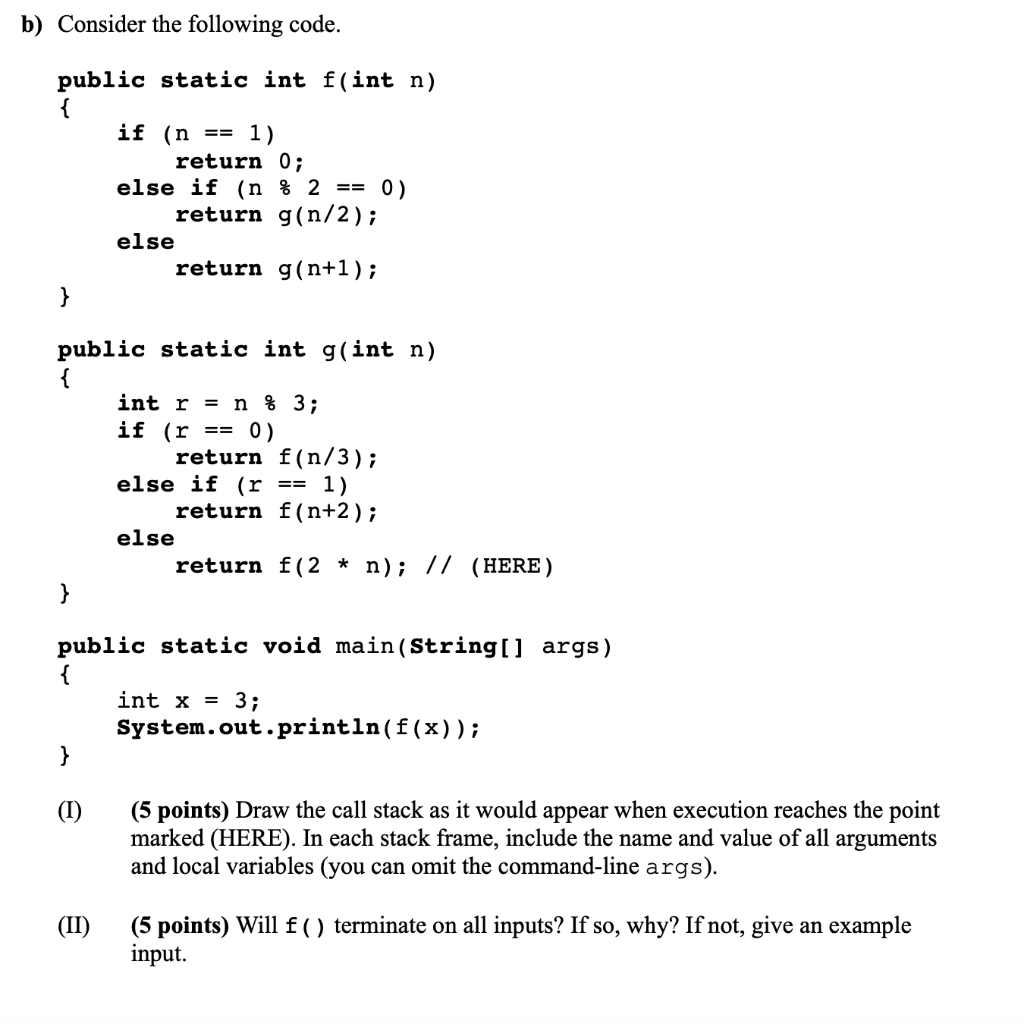b) Consider the following code. public static int f(int n) if (n == 1) return 0; else if (n % 2 == 0). return g(n/2); else return g(n+1); public static int g(int n) int r = n % 3; if (r == 0) return f(n/3); else if (r == 1) return f(n+2); else return f(2 * n); // (HERE) public static void main(String[] args) { int x = 3; System.out.println(f(x)); (1) (5 points) Draw the call stack as it would...

• ### Question 3: Given the following two code fragments [2 Marks] (i)Find T(n), the time complexity (as...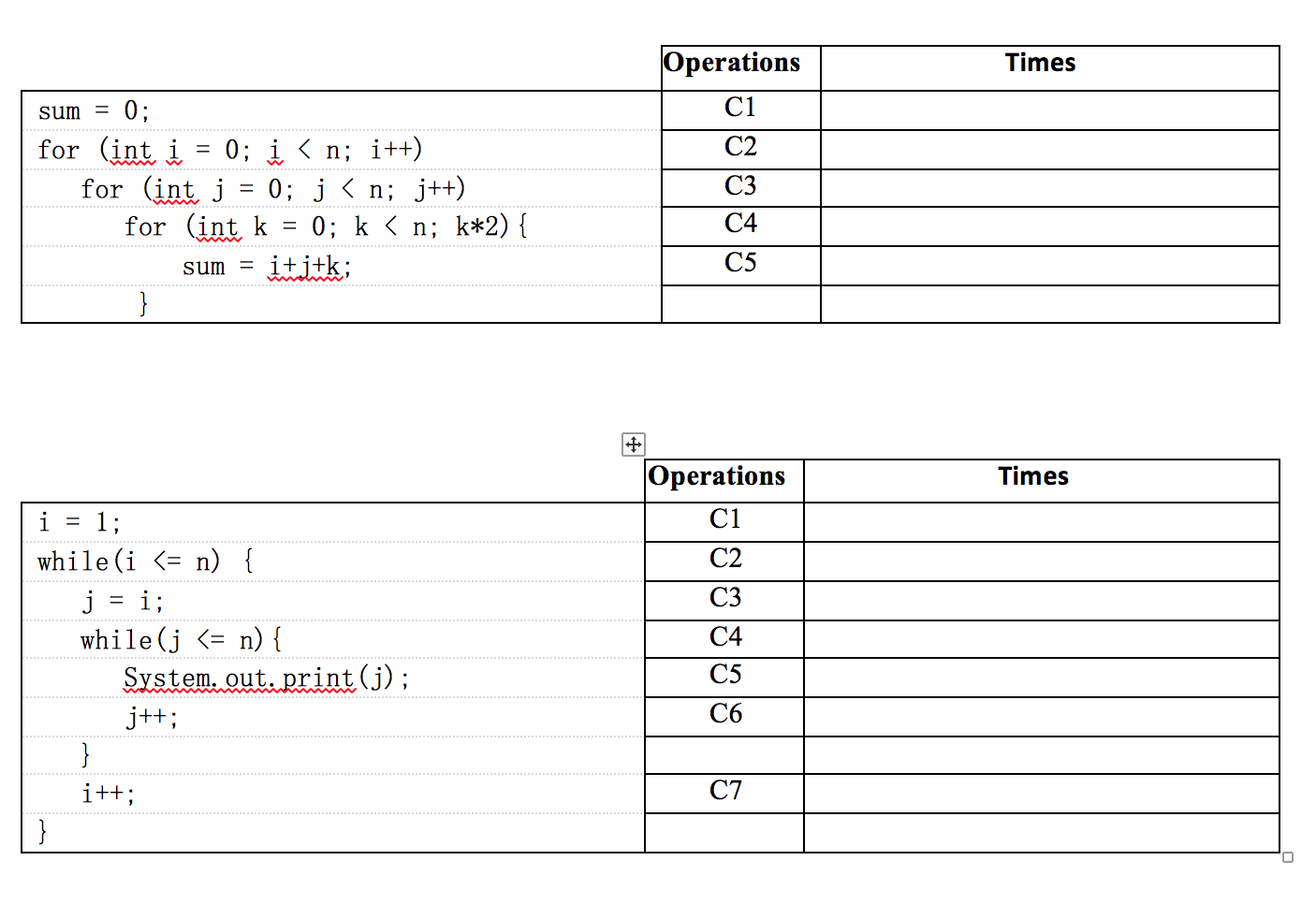Question 3: Given the following two code fragments [2 Marks] (i)Find T(n), the time complexity (as operations count) in the worst case? (ii)Express the growth rate of the function in asymptotic notation in the closest bound possible. (iii)Prove that T(n) is Big O (g(n)) by the definition of Big O (iv)Prove that T(n) is (g(n)) by using limits

• ### Question 1. (1 marks) The following procedure has an input array A[1..n] with n > 2...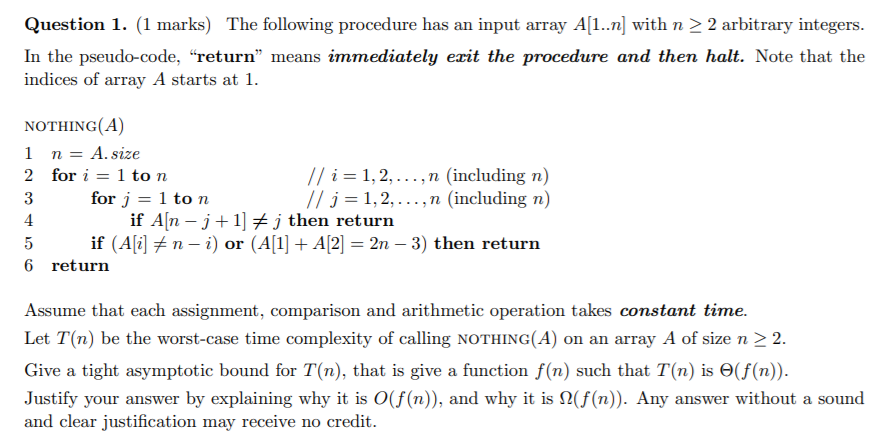Question 1. (1 marks) The following procedure has an input array A[1..n] with n > 2 arbitrary integers. In the pseudo-code, "return” means immediately erit the procedure and then halt. Note that the indices of array A starts at 1. NOTHING(A) 1 n = A. size 2 for i = 1 ton // i=1,2,..., n (including n) 3 for j = 1 ton // j = 1,2,...,n (including n) 4. if A[n - j +1] + j then return 5...

• ### Using the pseudocode answer these questions Algorithm 1 CS317FinalAlgorithm (A[O..n-1]) ito while i<n - 2 do...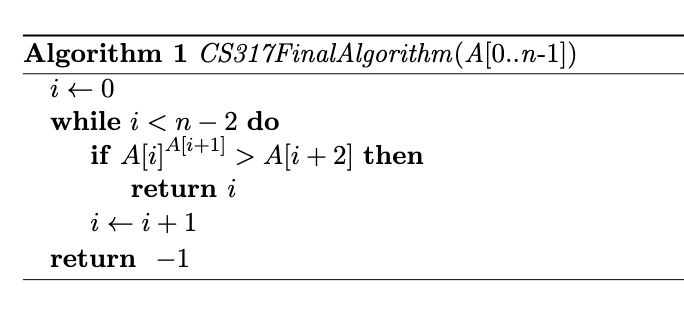Using the pseudocode answer these questions Algorithm 1 CS317FinalAlgorithm (A[O..n-1]) ito while i<n - 2 do if A[i]A[i+1] > A[i+2) then return i it i+1 return -1 6. Use limits to show that, for best case inputs, the asymptotic growth of the number of comparisons is (1). Show your work. 7. Use limits to show that, for worst case inputs, the asymptotic growth of the number of comparisons is O(n). Show your work.

• ### (V). Given the following algorithm, answer relevant questions. Algorithm 1 An algorithm 1: procedure WHATISTHIS(21,22,...,n: a...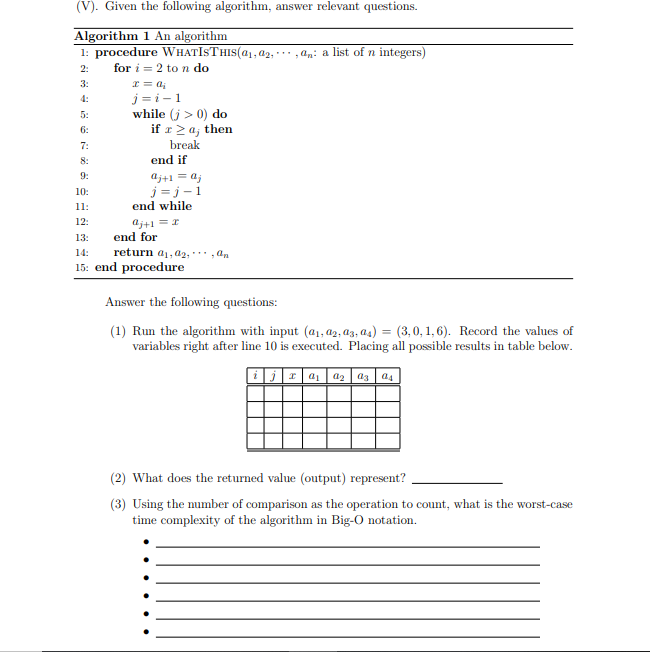(V). Given the following algorithm, answer relevant questions. Algorithm 1 An algorithm 1: procedure WHATISTHIS(21,22,...,n: a list of n integers) for i = 2 to n do c= j=i-1 while (j > 0) do if ra; then break end if 4j+1 = a; j= j-1 end while j+1 = 1 end for 14: return 0.02. 1, 15: end procedure Answer the following questions: (1) Run the algorithm with input (41, 02, 03, 04) = (3, 0, 1,6). Record the values...

• ### I only need help with question 5! Thank you! Question 4 4 pts Consider this variant...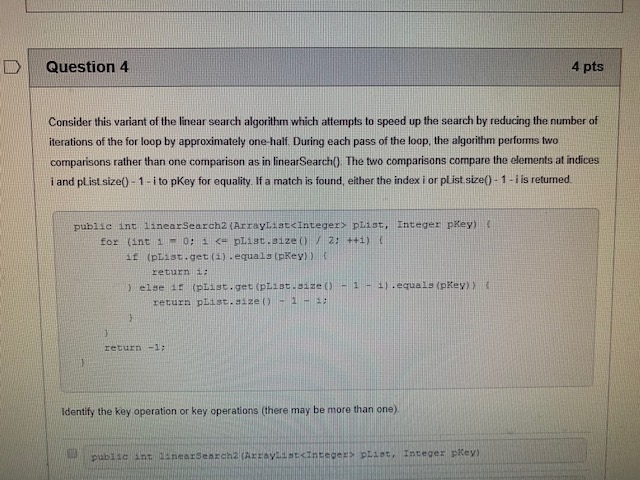I only need help with question 5! Thank you! Question 4 4 pts Consider this variant of the linear search algorithm which attempts to speed up the search by reducing the number of iterations of the for loop by approximately one-half. During each pass of the loop, the algorithm performs two comparisons rather than one comparison as in linear Search(). The two comparisons compare the elements at indices i and pList size() - 1-i to pKey for equality. If a...

• ### 3) [16 points totall Consider the following algorithm: int SillyCalc (int n) { int i; int Num, answer; if (n &lt...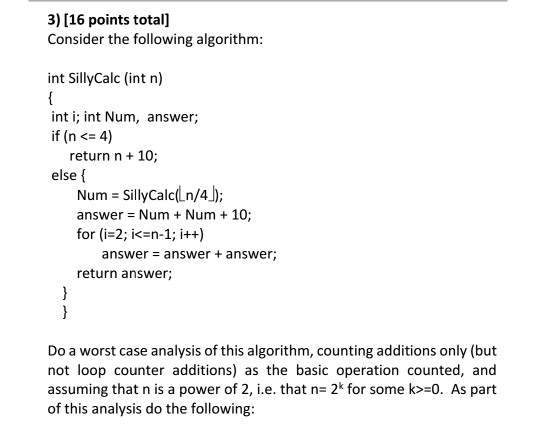3) [16 points totall Consider the following algorithm: int SillyCalc (int n) { int i; int Num, answer; if (n < 4) 10; return n+ else f SillyCalc(Ln/4) answer Num Num 10 for (i-2; i<=n-1; i++) Num + = answer + answer; answer return answer } Do a worst case analysis of this algorithm, counting additions only (but not loop counter additions) as the basic operation counted, and assuming that n is a power of 2, i.e. that n- 2*...